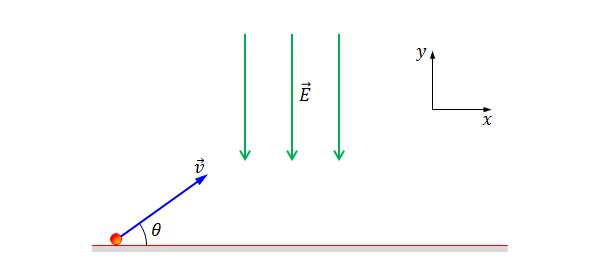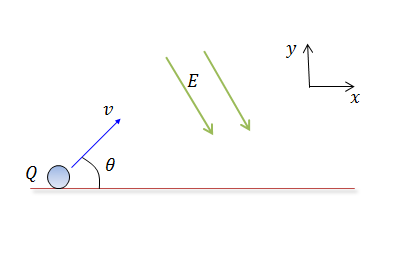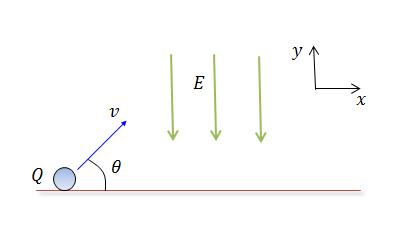Electricity and Magnetism

# Lorentz force (Electric fields)

An electron floats at rest above a sheet of charge with uniform charge density $\sigma$. What is $\sigma$ in $\mbox{C/m}^2$?

Details and assumptions

• The mass of the electron is $9.1 \times 10^{-31}~\mbox{kg}$.
• The electric charge on the electron is $-1.6 \times 10^{-19}~\mbox{C}$.
• The acceleration due to gravity is $-9.8~\mbox{m/s}^2$.
• The vacuum permittivity is $\epsilon_0=8.85 \times 10^{-12}~\mbox{F/m}$.
• If your answer is $\num{1.23e-4}$ submit 1.23E-4.A point particle with mass $m = 2 \text{ kg}$ and charge $Q = 33 \text{ C }$ is shot with speed $v = 8 \text{ m/s}$ at an angle of $\theta = 30^o$ with the ground. There is an electric field $\vec{E} = -22 \hat{y}\text{ N/C}$ heading perpendicularly to the ground. When the particle falls to the ground, the distance from the original point can be expressed as $R = \frac{a}{b} \text{ m},$ where $a$ and $b$ are coprime positive integers, what is the value of $a+b?$

Assume that $\sqrt{3} = 1.5$ and there is no gravitational field.A point particle with mass $m = 1\text{ kg}$ and charge $Q = 8 \text{ C }$ is shot with speed $v = 3 \text{ m/s}$ at an angle of $\theta = 30^\circ$ with the ground. There is an electric field $\vec{E} = 3\hat{x} - 6\hat{y} \text{ N/C},$ as shown in the above diagram. When the particle falls to the ground, the distance from the original point can be expressed as $R = \frac{a}{b} \text{ m},$ where $a$ and $b$ are coprime positive integers. What is the value of $a+b?$

Assume that $\sqrt{3} = 1.5 .$A point particle with mass $m = 6 \text{ kg}$ and charge $Q = 6 \text{ C }$ is shot with initial speed $v(0) = 6 \text{ m/s}$ at an angle of $\theta = 60^\circ$ with the ground. There is an electric field $\vec{E} = -6 \hat{y}\text{ N/C},$ as shown in the above diagram. When $t = \frac{3}{4}\text{ s},$ what is the speed of the particle ?

Assume that $\sqrt{3} = 1.5$A point particle with mass $m = 1 \text{ kg}$ and charge $Q = 9 \text{ C }$ is shot with speed $v = 6 \text{ m/s}$ at an angle of $\theta = 30^o$ with the ground. There is an electric field $\vec{E} = -9 \hat{y}\text{ N/C},$ as shown in the above diagram. If the maximum height can be expressed as $H = \frac{a}{b} \text{ m},$ where $a$ and $b$ are coprime positive integers, what is the value of $a+b?$

There is no gravitational field present.

×